### IMO Shortlist 2018 problem N6

Kvaliteta:
Avg: 5,0
Težina:
Avg: 8,0

Let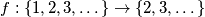$f : \{ 1, 2, 3, \dots \} \to \{ 2, 3, \dots \}$ be a function such that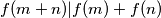$f(m + n) | f(m) + f(n)$ for all pairs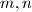$m,n$ of positive integers. Prove that there exists a positive integer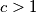$c > 1$ which divides all values of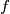$f$.

Izvor: https://www.imo-official.org/problems/IMO2018SL.pdf